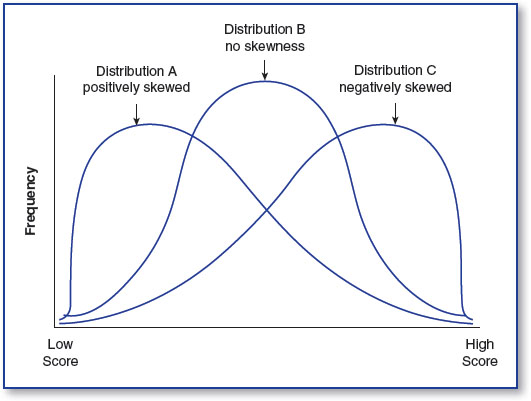In an increasingly data-driven world, it is more important than ever for students as well as professionals to better understand basic statistical concepts. 100 Questions (and Answers) About Statistics addresses the essential questions that students ask about statistics in a concise and accessible way. It is perfect for instructors, students, and practitioners as a supplement to more comprehensive materials, or as a desk reference with quick answers to the most frequently asked questions.

# What Are Skewness and Kurtosis, and How Do Distributions Differ on These Characteristics?

### What Are Skewness and Kurtosis, and How Do Distributions Differ on These Characteristics?

Not all distributions of scores are perfectly normal. In fact, while the distribution of many scores approaches a normal shape, many sets of scores are distributed in other ways.

Sometimes a distribution is skewed, showing a lack of symmetry such that one tail of the distribution is longer than the other tail. You can see an example of this in Figure 68.1.

### Figure 68.1 How a distribution of scores can be skewedIf the left-hand tail of a distribution is longer than the right, as when there are many higher values than lower values, then the distribution is negatively skewed. An example might be the distribution of measurements of height for 100 adults, ...

•••